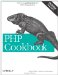# Recipe 4.11. Checking if an Element Is in an Array

#### 4.11.1. Problem

You want to know if an array contains a certain value.

#### 4.11.2. Solution

Use in_array( ):

`if (in_array(\$value, \$array)) {     // an element has \$value as its value in array \$array }`

#### 4.11.3. Discussion

Use in_array( ) to check if an element of an array holds a value:

`\$book_collection = array('Emma', 'Pride and Prejudice', 'Northhanger Abbey'); \$book = 'Sense and Sensibility'; if (in_array(\$book, \$book_collection) {     echo 'Own it.'; } else {     echo 'Need it.'; }`

The default behavior of in_array( ) is to compare items using the == operator. To use the strict equality check, ===, pass true as the third parameter to in_array( ):

`\$array = array(1, '2', 'three'); in_array(0, \$array);        // true! in_array(0, \$array, true);  // false in_array(1, \$array);        // true in_array(1, \$array, true);  // true in_array(2, \$array);        // true in_array(2, \$array, true);  // false`

The first check, in_array(0, \$array), evaluates to true because to compare the number 0 against the string tHRee, PHP casts three to an integer. Since three isn't a numeric string, as is 2, it becomes 0. Therefore, in_array( ) thinks there's a match.

Consequently, when comparing numbers against data that may contain strings, it's safest to use a strict comparison.

If you find yourself calling in_array( ) multiple times on the same array, it may be better to use an associative array, with the original array elements as the keys in the new associative array. Looking up entries using in_array( ) takes linear time; with an associative array, it takes constant time.

If you can't create the associative array directly but need to convert from a traditional one with integer keys, use array_flip( ) to swap the keys and values of an array:

`\$book_collection = array('Emma',                          'Pride and Prejudice',                          'Northhanger Abbey'); // convert from numeric array to associative array \$book_collection = array_flip(\$book_collection); \$book = 'Sense and Sensibility'; if (isset(\$book_collection[\$book])) {     echo 'Own it.'; } else {     echo 'Need it.'; }`

Note that doing this condenses multiple keys with the same value into one element in the flipped array.

Recipe 4.12 for determining the position of a value in an array; documentation on in_array( ) at http://www.php.net/in-array and array_flip( ) at the following address: http://www.php.net/array-flip.PHP Cookbook: Solutions and Examples for PHP Programmers
ISBN: 0596101015
EAN: 2147483647
Year: 2006
Pages: 445

Similar book on Amazon